Machine_Parts_Index

Shock Absorbers

These notes are very basic ..More detailed notes on shock absorber /isolation systems will be provided at a future date.

More details on Shock Absorber design are to be found in the links below the table

Introduction

The notes relate to simple examples of shock absorber/ isolation systems provided to minimise the risk of damage from impacts e.g. to prevent damage if a support screw fails and a load is accidentally released.

The detailed analysis of shock absorber/isolation systems is very complicated and involves assessment of the dynamic response of the equipment to different types of activating energy inputs.   The notes below relate only to illustrating the benefits of using shock absorbers to reduce the forces experienced by equipment to impacts.  The more complicated scenarios involving systems continuously operating and withstanding sudden changes of loading and acceleration e.g car suspension systems and aircraft landing gear, are outside of the scope of this work.

Moving objects have kinetic energy related to their velocity and their mass.   If the velocity of an object is significantly changed in a short time span e.g. it impacts on a stationary body, then high forces result.  These forces can be useful e.g a forging press using the kinetic energy to form metal.  However real life impact forces (shock loads) are generally very destructive and are avoided.

Kinetic energy increases in a direct ratio to the mass and to the velocity squared. The heavier the object, or the faster it travels, the more energy it has.   Methods of energy absorption include rubber buffers, metal springs, air springs, and hydraulic shock absorbers.   When the systems have to continuously operate under the influence of shock loads the shock isolation system generally include spring-dashpot isolation systems.   For simple shock absorber applications required to mitigate the effect of a single events then viscous dampers which dissipate the energy, as heat rise of a fluid, are often preferred.

In normal everyday life simple examples of shock absorber systems include crash helmets, steel toe caps in industrial boots, collapsible bumpers on cars, motor way barriers.

The notes below are general in nature provided to show the benefits of using shock absorbers.  For more detailed information - links are provided to shock absorber suppliers.

Shock Absorber types

There are a number of different methods of converting an impact /collision into relatively smooth cushioned contact..

 Metal Spring Rubber Buffer Hydraulic Dashpot Collapsing safety Shock Absorbers Pneumatic Cylinders Self compensating Hydraulic

Metal Springs ..

Simply locating metal springs to absorb the impact loads are a low cost method of reducing the collision speed and reducing the shock loading.  They are able to operate in very arduous conditions under a wide range of temperatures.  These devices have high stopping forces at end of stroke.   Metal springs store energy rather than dissipating it .  If metal sprint type shock absorbers are used then measures should be provided to limit oscillations ---Metal spring are often used with viscous dampers .

There are a number of different types of metal springs includine helical springs, bellville washers(cone-springs), leaf springs, ring springs, mesh springs etc etc.   Each spring type has its own operating characteristics.

Elastomeric Shock absorbers..

These are a low cost options for reducing the collision speed and reducing the shock loading and providing system damping.  They are conveniently moulded to suitable shapes.   These devices have high stopping forces at end of stroke with significant internal damping.    Elastomeric dampers are very widely used because of the associated advantages of low cost and mouldability together with performance benefits.  The inherent damping of elastomers is useful in preventing excessive vibration amplitude at resonance - much reduced compared to metal springs.

However elastomer based shock absorbers are limited in being affected by high and low temperatures. and are subject to chemical attack.  Silcone rubber is able to provide reasonable mechanical properties between temperatures of -50O to +180O deg. C- most other elastomers have inferior temperature tolerance.

Hydraulic Dashpot

This type of shock absorber is based on a simple hydraulic cylinder.  As the piston rod is moved hydraulic fluid is forced through an orifice which restricts flow and consequently provides a controlled resistance to movement of the piston rod.

With only one metering orifice the moving load is abruptly slowed down at the start of the stroke.   The braking force rises to a very high peak at the start of the stroke and then falls away rapidly.  On completion of the stroke the system is stable - the energy being dissipated in the hydraulic fluid as heat.  These type of shock aborbers are provided with springs sufficient to return the actuator to its initial position after the impacting load is removed.

Collapsing Safety Shock Absorbers

These are single use units which are generally specially designed for specific duties.  They are designed such that at impact they collapse and the impact energy is absorbed as the materials distort in their inelastic/yield range.   They therefore are more compact compared to devices based on deflections within their elastic range.

Air ( Pneumatic) Springs

These devices use air as the resilient medium . Air has a high energy storage capacity compared to metal or elastomer materials.  For duties with high loads and deflections the air spring is generally far more compact that the equivalent metal or elastomer device.

Due to the compressibility of air these have a sharply rising force characteristic towards the end of the stroke.   The majority of the energy is absorbed near the end of the stroke.  The force on an air cylinder buffer is determined by the relationship PVn = constant.

Air springs require more maintenance than meal or elastomer based springs and the temperature range is restricted compared to metal springs.

Self compensating Hydraulic

These devices are similar to the hydraulic dashpot type except that a number of orifices are provided allowing different degrees of restriction throughout the stroke.

These devices are engineered to bring the moving load is smoothly and gently to rest by a constant resisting force throughout the entire shock absorber stroke.   The load is decelerated with the lowest possible force in the shortest possible time eliminating damaging force peaks and shock damage to machines and equipment.   These type of shock absorbers are provided with springs sufficient to return the actuator to its initial position after the impacting load is removed.

Shock Absorber Comparison

These notes identify the high force that can result from and impact and the show the reduction in force by use of a spring and a compensating hydraulic shock absorber.  The example is provides as a general illustration and is very much simplified.

Force resulting from impact with No shock absorber included

Considering a very simple duty of dropping a 1 kg load through 0,5 m onto a machine element represented by a short steel column 0,1m dia by 0,2m long made form steel.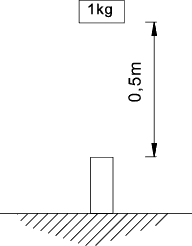The stiffness of the column k = AE / l.

A = 0,00785m2
E = 21x1010 Pa ( N/m2 )
l = 0,2m

The stiffness of the column k is the Load /unit deflection is calculated as..

k = 0,00785  .  21  .  1010 / 0,2 = 0,825 . 1010 N/m

To calculate the maximum force resulting from the dropped load assuming conservation of energy.
The strain energy absorbed by the column = the Potential energy absorbed from the dropped load. The potential energy of the load = E 1

E 1 = M.g.h = 1 .  9,81  .  0,5 = 4.905 Nm.

This equals the strain energy absorbed by the load at impact

The strain energy absorbed = Pmaxδmax /2 = Pmax2 / 2 k
( δmax = Pmax /k )

Note: This is a transient (shock load) with an associated transient deflection of the impact surface.
In practice is would be less than the value calculate by this equation because of energy losses due to internal friction in the material. Reference Impact

Therefore to calculate the maximum force developed Pmax

Pmax = Sqrt (2.E1.k) = Sqrt (2  .  4,905  .  0,825 . 1010 ) = 284 kN
δmax = Pmax / k = 284. 103 / 0,825 . 1010 m = 34 . 10-6 m

This value can also be determined in a similar manner using the principles established on page   Impact Loading

 The stress assuming the load is applied gradually = σst = Mg/A σst = 1 .  9,81  /  0,00784 = 1249 N/m2 The static deflection δst = W/k = Mg /k δst = 1  .  9,81  / ( 0,825   .x  1010 ) =11,89  .  10-10m With reference to   Impact Loading   The Maximum stress = obtained from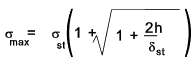The maximum stress can be obtained from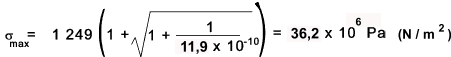The maximum (transient ) force experienced as a result of the dropped load. Pmax= σmax.A = 284 kN

Maximum force Resulting From Use of Spring

If a spring with a stroke of 0,1m is located on the top surface as shown below.  The spring is rated to absorb all of the energy resulting from the dropped load.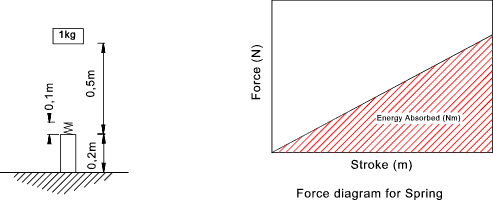The resulting maximum force is determined as follows.

Energy to be absorbed = E1 = M.g.h. = 4.905 Nm
Strain energy of spring = Fmax.δspring / 2
Therefore Maximum force Fmax = 2.M.g.h / δspring = 98.1N

Use of the spring has reduced the maximum force by a factor of 3000.   However the spring is now exerting an upward force which will cause the load to rebound upwards.  Detailed analysis of the system response is required to arrive at the total motion history of this event

Maximum force Resulting From Use A compensating Hydraulic Shock absorber

If a Shock absorber with a stroke of 0,1m is located on the top surface as shown below
It is assumed that the shock absorber is designed to provide a constant decelaration force throughout its stroke..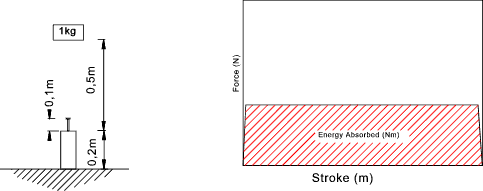The resulting maximum force is determined as follows.

Energy to be absorbed = E1 = Mgh. = 4.905 Nm
Energy to be dissipated in the shock absorber = F . δsh_ab
Therefore Maximum force = M.g. h/δsh_ab = 49.05 N

The energy has been dissipated in heating up the hydraulic fluid in the shock absorber. When the load has come to rest the system is in a stable state.  The maximum force transmitted to the column during impact is significantly less than that experienced by without the shock absorber.

Shock absorber duty requirement

The capacity of a shock absorber is determined by calculating the energy to be absorbed.   The calculation of the energy absorbed is shown in the notes below which illustrate some of the different applications.

Free falling mass;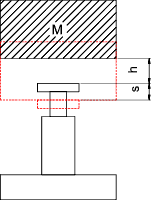Assessing the shock absorber requirements.

The energy to be absorbed at first impact with the shock absorber E 1= Mgh.

E 1= Mgh.

The additional energy to be absorbed in the shock absorber stroke

E 2= Mgs.

The total energy to be absorbed by the shock absorber .

E t= E 1+ E 2

The total energy to be absorbed/hour by the shock absorber. (n = number of operations per hour).

E th= E tn

The velocity at impact on the shock absorber.

v d = Sqrt(2gh)

The equivalent weight to be supported by the shock absorber

Me= 2M / v d2

Horizontal moving mass;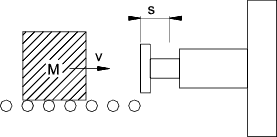Assessing the shock absorber requirements.

Assuming moving friction = 0
The energy to be absorbed at first contact with the shock absorber E 1.

E 1= Mv2/ 2

The additional energy to be absorbed in the shock absorber stroke .

E 2= 0

The total energy to be absorbed by the shock absorber

E t= E 1+ E 2

The total energy to be absorbed/hour by the shock absorber. (n = number of operations per hour)

E th= E tn

The velocity at impact on the shock absorber

v d = v

The equivalent weight to be supported by the shock absorber

Me= M

Horizontal moving mass with constant propelling force;

Assuming moving friction = 0
v = velocity at initiation of propelling force.
H = distance over which propelling force is operating.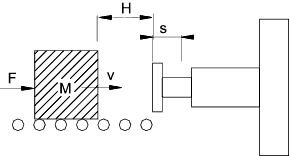The energy to be absorbed at first contact with the shock absorber E 1.

E 1= Mvd2 /2

The additional energy to be absorbed in the shock absorber stroke .

E 2= Fs

The total energy to be absorbed by the shock absorber.

E t= E 1+ E 2

The total energy to be absorbed/hour by the shock absorber. (n = number of operations per hour).

E th= E tn

The velocity at impact on the shock absorber.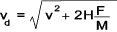The equivalent weight to be supported by the shock absorber.

Me= 2.Et / v d2

Mass Moving down incline

Assuming moving friction = 0
Assuming mass starts from a resting position.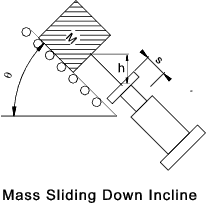The energy to be absorbed at first contact with the shock absorber E 1

E 1= Mgh = Mvd2 / 2.

The additional energy to be absorbed in the shock absorber stroke.
(No Driving Force F = 0)

E 2=M g s sin θ

The total energy to be absorbed by the shock absorber.

E t= E 1+ E 2

The total energy to be absorbed/hour by the shock absorber. (n = number of operations per hour).

E th= E tn

The velocity at impact on the shock absorber.

vd = Sqrt (2 g h)

The equivalent weight to be supported by the shock absorber .

Me= 2.Et / v d2

Mass Moving down incline with downward force

Assuming moving friction = 0
Assuming mass starts from a resting position.
h = Vertical distance over which propelling force is operating.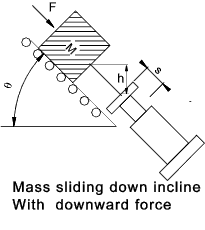The energy to be absorbed at first contact with the shock absorber E 1.

E 1= (F sin θ + Mg)h = Mvd2 / 2.

The additional energy to be absorbed in the shock absorber stroke
propelling Force Down incline .

E2 = (F + Mg sinθ) � s

The total energy to be absorbed by the shock absorber.

E t= E 1+ E 2

The total energy to be absorbed/hour by the shock absorber. (n = number of operations per hour).

E th= E tn

The velocity at impact on the shock absorber.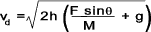The equivalent weight to be supported by the shock absorber .

Me= 2.Et / v d2

Mass Moving up incline due to upward force

Assuming moving friction = 0
Assuming mass starts from a resting position

h = Vertical distance over which propelling force is operating.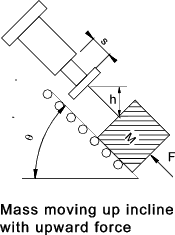The energy to be absorbed at first contact with the shock absorber E 1.

E 1= (F sin θ - Mg)h =Mvd2 /2

The additional energy to be absorbed in the shock absorber stroke.

E2 = (F - M g sinθ) � s

The total energy to be absorbed by the shock absorber .

E t= E 1+ E 2

The total energy to be absorbed/hour by the shock absorber. (n = number of operations per hour).

E th= E tn

The velocity at impact on the shock absorber.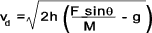The equivalent weight to be supported by the shock absorber .

Me= 2.Et / v d2

Swinging Mass Without Propelling Torque

The swinging mass is assumed to be swinging on a horizontal plane..
If the mass is swinging in a vertical plane then the effects of gravity are not considered.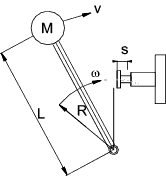The energy to be absorbed at first contact with the shock absorber E 1

E 1= Mv2/2 = Iω2 /2

The additional energy to be absorbed in the shock absorber stroke .

E2 = 0

The total energy to be absorbed by the shock absorber .

E t= E 1+ E 2

The total energy to be absorbed/hour by the shock absorber. (n = number of operations per hour).

E th= E tn

The velocity at impact on the shock absorber

vd = vR/L = ωR

The equivalent weight to be supported by the shock absorber .

Me= 2.Et / v d2

Swinging Mass With constant Propelling Torque T

The swinging mass is assumed to be swinging on a horizontal plane.
If the mass is swinging in a vertical plane then the effects of gravity are not considered .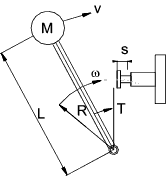The energy to be absorbed at first contact with the shock absorber E 1.

E 1= Mv2/2 = Iω2 /2

The additional energy to be absorbed in the shock absorber stroke.

E2 = Ts /R

The total energy to be absorbed by the shock absorber .

E t= E 1+ E 2

The total energy to be absorbed/hour by the shock absorber. (n = number of operations per hour).

E th= E tn

The velocity at impact on the shock absorber.

vd = vR/L = ωR

The equivalent weight to be supported by the shock absorber.

Me= 2.Et / v d2

 Links to Spring Design tpcdayton ...Anti-Vibration Mountings Shock Absorbers ...Notes Briely reviewing the different types of shock absorbers Monroe ..Shock absorbers as used for automotive applications Ace Controls ...Shock absorber supplier catalogue including required calculations Enertrols ...Shock absorber supplier USA based - mostly imperial units Enidine Shock Absorbers ...Some theory and animations

Machine_Parts_Index